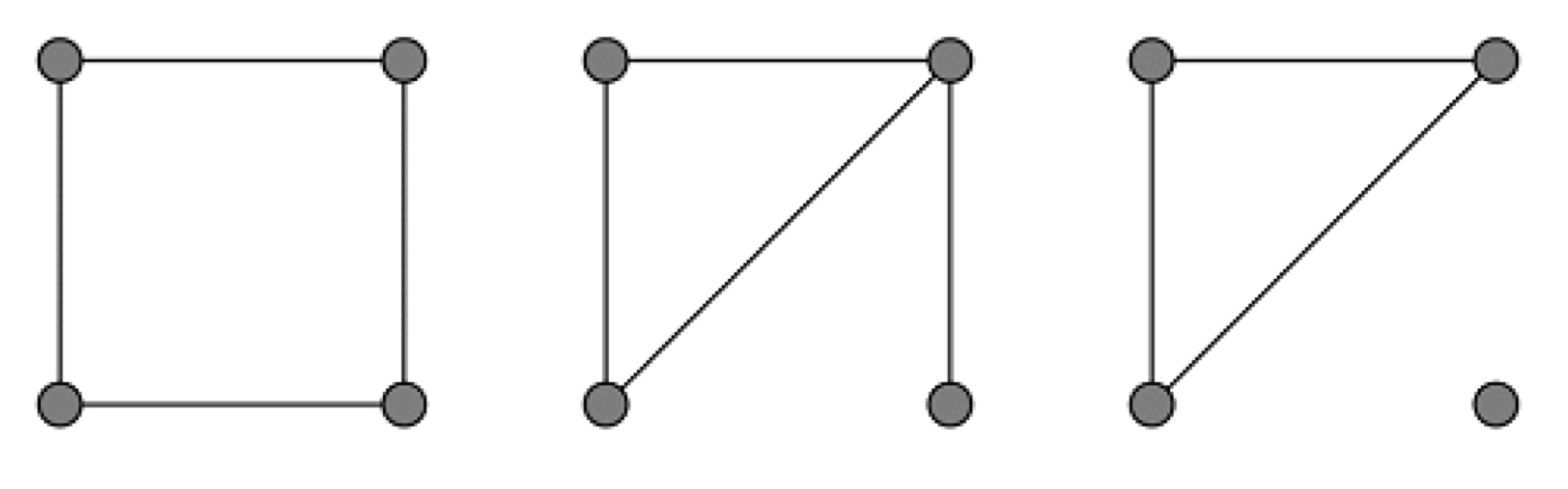시간 제한메모리 제한제출정답맞힌 사람정답 비율
2 초 (추가 시간 없음) 256 MB222100.000%

## 문제

We call a simple graph good if each component of the graph has at most one cycle.

Your task is to count the number of edges belonging to one cycle for all the good graphs with $n$ labeled vertices.

In order to avoid calculations of huge integers, report the sum of the number of edges modulo $p$ instead.

## 입력

There are multiple test cases. The first line of the input contains two integers $T$ and $p$ ($1 \le T \le 3000$, $1 \le p \le 2^{30}$), indicating the number of test cases and the modulus. For each test case:

The first line contains the only integer $n$ ($1 \leq n \leq 3000$).

## 출력

For each test case, output the sum of the numbers of edges modulo $p$ in one line.

## 예제 입력 1

7 998244353
1
2
3
4
5
6
7


## 예제 출력 1

0
0
3
60
1050
19380
393750


## 노트

There are three types of good graphs having four labeled vertices in which cycles exist.The numbers of these graphs are $3$, $12$ and $4$ respectively. Consequently, the sum of the numbers of edges is $3 \times 4 + 12 \times 3 + 4 \times 3 = 60$.